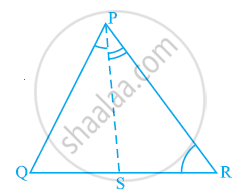# In the given figure, PR > PQ and PS bisects ∠QPR. Prove that ∠PSR >∠PSQ. - Mathematics

In the given figure, PR > PQ and PS bisects ∠QPR. Prove that ∠PSR >∠PSQ.#### Solution

As PR > PQ,

∴ ∠PQR > ∠PRQ (Angle opposite to larger side is larger) ... (1)

PS is the bisector of ∠QPR.

∴∠QPS = ∠RPS ... (2)

∠PSR is the exterior angle of ΔPQS.

∴ ∠PSR = ∠PQR + ∠QPS ... (3)

∠PSQ is the exterior angle of ΔPRS.

∴ ∠PSQ = ∠PRQ + ∠RPS ... (4)

Adding equations (1) and (2), we obtain

∠PQR + ∠QPS > ∠PRQ + ∠RPS

⇒ ∠PSR > ∠PSQ [Using the values of equations (3) and (4)]

Concept: Inequalities in a Triangle
Is there an error in this question or solution?
Chapter 7: Triangles - Exercise 7.4 [Page 132]

#### APPEARS IN

NCERT Class 9 Maths
Chapter 7 Triangles
Exercise 7.4 | Q 5 | Page 132

Share